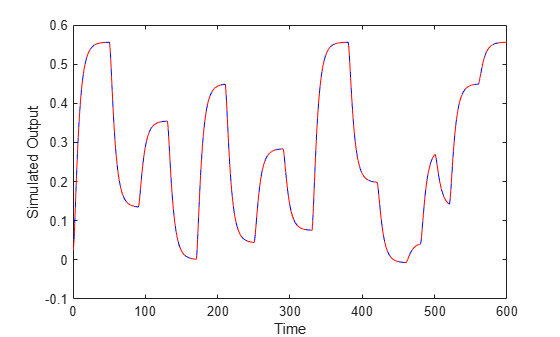Documentation

How the Software Computes Hammerstein-Wiener Model Output

This topic describes how the software evaluates the output of nonlinearity estimators and uses this output to compute the response of a Hammerstein-Wiener model.

Evaluating Nonlinearities (SISO)

Evaluating the output of a nonlinearity for a input u requires that you first extract the input or output nonlinearity from the model:

F = M.InputNonlinearity;
H = M.OutputNonlinearity;

Evaluate F(u):

w = evaluate(F,u)

where u is a scalar representing the value of the input signal at a given time.

You can evaluate output at multiple time instants by evaluating F for several time values simultaneously using a column vector of input values:

w = evaluate(F,[u1;u2;u3])

Similarly, you can evaluate the value of the nonlinearity H using the output of the linear block x(t) as its input:

y = evaluate(H,x)

Evaluating Nonlinearities (MIMO)

For MIMO models, F and H are vectors of length nu and ny, respectively. nu is the number of inputs and ny is the number of outputs. In this case, you must evaluate the predicted output of each nonlinearity separately.

For example, suppose that you estimate a two-input model:

M = nlhw(data,[nb nf nk],[wavenet;poly1d],'saturation')

In the input nonlinearity:

F = M.InputNonlinearity
F1 = F(1);
F2 = F(2);

F is a vector function containing two elements: F=[F1(u1_value);F2(u2_value)], where F1 is a wavenet object and F2 is a poly1d object. u1_value is the first input signal and u2_value is the second input signal.

Evaluate F by evaluating F1 and F2 separately:

w1 = evaluate(F(1),u1_value);
w2 = evaluate(F(2),u2_value);

The total input to the linear block, w, is a vector of w1 and w2 (w = [w1 w2]).

Similarly, you can evaluate the value of the nonlinearity H:

H = M.OutputNonlinearity;

Simulation of Hammerstein-Wiener Model

This example shows how the software evaluates the simulated output by first computing the output of the input and output nonlinearity estimators.

Estimate a Hammerstein-Wiener model.

estData = iddata(y,u,0.2);
M = nlhw(estData,[1 5 3],'pwlinear','poly1d');

Extract the input nonlinearity, linear model, and output nonlinearity as separate variables.

uNL = M.InputNonlinearity;
linModel = M.LinearModel;
yNL = M.OutputNonlinearity;

Simulate the output of the input nonlinearity estimator.

Input data for simulation

u = estData.u;

Compute output of input nonlinearity

w = evaluate(uNL,u);

Compute response of linear model to input w and zero initial conditions.

x = sim(linModel,w);

Compute the output of the Hammerstein-Wiener model M as the output of the output nonlinearity estimator to input x.

y = evaluate(yNL,x);

The previous set of commands are equivalent to directly simulating the output of M using the sim command.

ysim = sim(M,u);

Plot y and ysim, the manual and direct simulation results, respectively.

time = estData.SamplingInstants;
plot(time,y,'b',time,ysim,'--r');
xlabel('Time');
ylabel('Simulated Output')The plot indicates that y and ysim are the same.